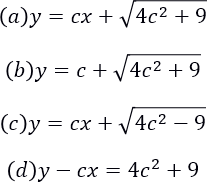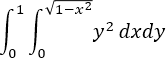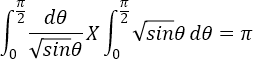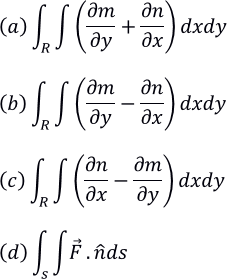MORE IN Engineering Maths 2
Total marks: --
Total time: --
INSTRUCTIONS
(1) Assume appropriate data and state your reasons
(2) Marks are given to the right of every question
(3) Draw neat diagrams wherever necessary

1 (a) (i) We say that the given differential equation is solvable for x , if it is possible to express in terms of:
(a) x and y
(b) x and p
(c) y and p
(d) x,y and p
1 M
1 (a) (ii) The general solution of P2-7P+12=0 is:
(a) (y+3x-c)(y+4x-c) = 0
(b) (y-3x-c)(y-4x-c) = 0
(c) (y-4x)(y+3x) = 0
(d) none of these
1 M
1 (a) (iii) The general solution of the equation y = 3x + logP is:
(a) y=3x+3+cey
(b) y=3x+log(3+cey)
(c) y+3x=3+cey
(d) None of these
1 M
1 (a) (iv) The general solution of the equation (y-Px)2=4P2+9 is:1 M
1 (b) Solve the following: p2+2py cotx=y2
5 M
1 (c) Solve the following: p2+ 4x5p-12x4y=0. Obtain the singular solution also.
5 M
1 (d) Solve the equation (px-y)(px+y)=2p by reducing into Clairaut's form, taking the substitution X=x2, Y=y2
6 M

2 (a) (i) P.I. of y''-3y'+2y=12 is:
(a) 6
(b) y=c1ex+c2e2x
(c) 1/12
(d) 1/6
1 M
2 (a) (ii) The complementary function of (D4 - a4 )y = 0 is:
(a) y = c1eax+c2e-ax+c3cosx+c4sinx
(b) y = c1e-ax+c2eax
(c) y = c1eax+c2e-ax+c3cosax+c4sinax
(d) none of these

1 M
2 (a) (iii) If F(D)=D2+5, sin2x/f(D)=?
(a) -cos2x/2
(b) cos2x/2
(c) sin2x
(d) cos2x
1 M
2 (a) (iv) The solution of the differential equation y"-3y'+2y=e3x is?
(a)y=c1e-x+c2e-2x+0.5e3x
(b) y=c1ex+c2e2x+0.5e3x
(c) y=c1e-x+c2e2x+0.5e3x
(d) y=c1e-x+c2e2x+0.5e-3x
1 M
2 (b) Solve : (D-2)2y=8(e2x+sin2x)
5 M
2 (c) Solve: y"-2y'+y=xcosx
5 M
2 (d) Solve: dx/dt-2y=cos2t; dy/dt+2x=sin2t; given that x=1, y=0 at t=0.
6 M

3 (a) (i) The Wronkian of x and ex is:
(a) ex(1-x)
(b) xex
(c) e-x(x-1)
(d) ex(x-1)
1 M
3 (a) (ii) In the equation dx/dt+y=sint+1; dy/dt+x=costt; if y=sint+1+e-t, then x=?
(a) 0
(b) e-t
(c) xe-t
(d) et
1 M
3 (a) (iii) In homogenous linear differential equation whose auxiliary equation has roots 1,-1 is:
(a) y"+y=0
(b) x2y"-xy'-y=0
(c) x2y"+xy'-y=0
(d) y"-y'=0
1 M
3 (a) (iv) The solution of x2y"+xy'=0 is:
(a) y=c1+c2logx
(b) y=alogx+6
(c) y=et
(d) y=e-t
1 M
3 (b) Using the method of varioation of parameters, solve: y"+4y=tan2x
5 M
3 (c) Solve: (1+x)2y"+(1+x)y'+y=sin2[log(1+x)]
5 M
3 (d) Solve by Frobenius method, the eqaution 4xd2y/dx2+2dy/dx+y=0
6 M

4 (a) (i) The solution of ∂2z/∂y2=sin(xy) is:
(a) z = -x2sin(xy) + yf(x) +φ(x)
(b) z = cos(xy)/x2 + yf(x) +φ(x)
(c) z = -sin(xy)/x2 + yf(x) +φ(x)
(d) None of these
1 M
4 (a) (ii) A solution of (y-z)p+(z-x)q = x-y is:
(a) x2+y2+z2=f(x+y+z)
(b) x2-y2-z2=f(x-y+z)
(c) x2-y2-z2=f(x-y-z)
(d) none of these
1 M
4 (a) (iii) The partial differential equation obtained from z=ax+by+ab by eliminating a and b is:
(a)z=px+qy
(b)z=px+qy+pq
(c)z=px+qy-pq
(d)z=px-qy-pq
1 M
4 (a) (iv) The partial differential equtaion obtained from z = f(x+y)+g(x-y) by eliminating the arbitrary functions is:
(a) r+t=0
(b) r-t=0
(c) r-a2t=0
(d) r+a2t=0
1 M
4 (b) Solve: ∂2z/∂x2+z=0,given that when x=0,z=ey and ∂z/∂x=1
5 M
4 (c) Solve: (x2-yz)p+(y2-zx)q = z2-xy
5 M
4 (d) Solve by the method of variables: 4∂u/∂x+∂u/∂y=3u, given that u(0,y)=2e5y
6 M

5 (a) (i) The value of ∫1213 xy2dxdy is:
(a) 0
(b) 1
(c) 13/2
(d) 13
1 M
5 (a) (ii) The integral ∫0x e-y/y dxdy after changing the order of integration is:
(a) ∫00y e-y/y dxdy
(b) ∫0xxy e-y/y dxdy
(c) ∫00 e-y/y dxdy
(d) ∫00x e-y/y dxdy
1 M
5 (a) (iii) B(1/2,1/2) = ?
(a) √π
(b) √π/2
(c) 3.1416
(d) -π
1 M
5 (a) (iv) In terms of Beta function ∫0π/2 sin7θ·√(cosθ)dθ = ?
(a) β(4, 3/4)
(b) 1/2 β(4, 3/4)
(c) β(2, 3/2)
(d) 1/2 β(2, 3/2)
1 M
5 (b) Change the order of integration and hence evaluate the same in:5 M
5 (c) Evaluate ∫0a0x0x+y ex+y+z dzdydx
5 M
5 (d) Show that:6 M

6 (a) (i) In Green's theorem in the plane ∮ mdx+ndy = ?1 M
6 (a) (ii) The area of the ellipse x2/a2 +y2/b2 =1 by employing Green's theorem is:
(a) 0
(b) 1
(c) π
(d) πab
1 M
6 (a) (iii) The necessary and sufficient condition that the line integral ∫L F.dR for every closed curve C is:
(a) curl F=0
(b) div F=0
(c) curl F≠0
(b) div F≠0
1 M
6 (a) (iv) If V is the volume bounded by a surface S and F is continuously differentiable vector function then ∫ ∫v ∫ divFdv = ?
(a) ∮eF·dr
(b) ∫S ∫F·n^ds
(c) ∫S∫(∇×F) n^ds
(d) none of these
1 M
6 (b) If F=2xyi+yz2j+xzk and S is the rectangular parallelepiped bounded by  x=0, y=0, z=0, x=2, y=1, z=3, evaluate ∫S∫ F·n^ds
5 M
6 (c) Using Green's Theorem, evaluate ∫C[(y-sinx)dx + cosxdy] ,where C is the plane enclosed by the lines y=0, x=π/2 and y=2x/π
5 M
6 (d) Verify Stoke's theorem for F=(x2+y2)i-2xyj taken around the rectangle bounded by the lines x=±a, y=0, y=b
6 M

7 (a) (i) L{e2(t-1)} = ?
(a) 1/(s-2)
(b) e-2/(s-2)
(c) 1/(s+2)
(d) e-2/(s+2)
1 M
7 (a) (ii) L{t1⁄2 } = ?
(a)  √π/s3⁄2
(b)  2√π/s3⁄2
(c)  √π/(2√s)
(d)  √π/ 2s3⁄2
1 M
7 (a) (iii) L{sint/t} = ?
(a) π/2 + tan-1s
(b) π/2 - cot-1s
(c) cot-1s
(d) tan-1s
1 M
7 (a) (iv) L{δ(t+2)} = ?
(a) e-as
(b) e2s
(c) e2s
(d) eas
1 M
7 (b) Find the value of ∫0t3e-tsint dt using Laplace transforms
5 M
7 (c) Draw the graph of the periodic function and find its Laplace transform
f(t) = t, 0<t<π
f(t) = π-t, π<t<2π
5 M
7 (d) Prove that L{δ(t-a)} = e-as
6 M

8 (a) (i) L-1{1/(4s2-36)} = ?
(a) (cosh6t)/4
(b) (sin3t)/12
(c) (cosh3t)/6
(d) (sinh3t)/12
1 M
8 (a) (ii) L-1{(1+e-3s)/s2} = ?
(a) t + (t-3)u(t-3)
(b) (t-3)u(t-3)
(c) t - (t-3)u(t-3)
(d) t+(t+3)u(t+3)
1 M
8 (a) (iii) L-1{cot-1(s/a)} = ?
(a) (sint)/t
(b) (sinat)/t
(c) (sinhat)/t
(d) (sinht)/t
1 M
8 (a) (iv) L [∫0t f(u)·g(t-u)du] = ?
(a) f(t)g(t)
(b) f(s)g(s)
(c) f(s)-g(s)
(d) f(s)/g(s)
1 M
8 (b) Find L-1{(5s+3)/(s-1)(s2+2s+5)}
5 M
8 (c) Apply Convolution Theorem to evaluate: L-1{s2/(s2+a2)(s2+b2) }
5 M
8 (d) Solve(D3-3D2+3D-1)y = t2et; y(0)=1, y'(0)=0, y''(0) = -2 by Laplace transform method.
6 M

More question papers from Engineering Maths 2